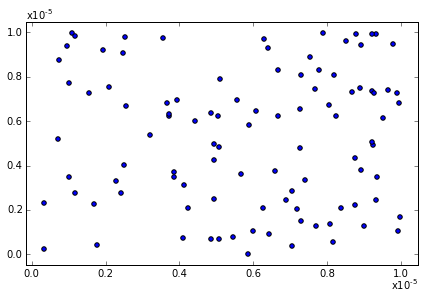In :
%matplotlib inline

In :
def format_exponent(ax, axis='y'):

# Change the ticklabel format to scientific format
ax.ticklabel_format(axis=axis, style='sci', scilimits=(-2, 2))

# Get the appropriate axis
if axis == 'y':
ax_axis = ax.yaxis
x_pos = 0.0
y_pos = 1.0
horizontalalignment='left'
verticalalignment='bottom'
else:
ax_axis = ax.xaxis
x_pos = 1.0
y_pos = -0.05
horizontalalignment='right'
verticalalignment='top'

# Run plt.tight_layout() because otherwise the offset text doesn't update
plt.tight_layout()
##### THIS IS A BUG
##### Well, at least it's sub-optimal because you might not
##### want to use tight_layout(). If anyone has a better way of
##### ensuring the offset text is updated appropriately

# Get the offset value and the position of the offset text
offset = ax_axis.get_offset_text().get_text()
pos = ax_axis.get_offset_text().get_position()

if len(offset) > 0:
# Get that exponent value and change it into latex format
minus_sign = u'\u2212'
expo = np.float(offset.replace(minus_sign, '-').split('e')[-1])
offset_text = r'x$\mathregular{10^{%d}}$' %expo

# Turn off the offset text that's calculated automatically
ax_axis.offsetText.set_visible(False)

# Add in a text box at the top of the y axis
ax.text(x_pos, y_pos, offset_text, transform=ax.transAxes,
horizontalalignment=horizontalalignment,
verticalalignment=verticalalignment)
return ax

In :
def get_min_max(x, pad=0.05):
'''
Find min and max values such that
all the data lies within 90% of
of the axis range
'''
r = np.max(x) - np.min(x)
x_min = np.min(x) - pad * r
x_max = np.max(x) + pad * r
return x_min, x_max

In :
import matplotlib.pylab as plt
import numpy as np

# Create a figure and axis
fig, ax = plt.subplots()

# Plot 100 random points that are very small
x = np.random.rand(100)/100000.0
y = np.random.rand(100)/100000.0
ax.scatter(x, y)

x_min, x_max = get_min_max(x)
y_min, y_max = get_min_max(y)

ax.set_xlim(x_min, x_max)
ax.set_ylim(y_min, y_max)

ax = format_exponent(ax, axis='x')
ax = format_exponent(ax, axis='y')

# And show the figure
plt.show()In [ ]:


In [ ]: